# Euclidean ring

(diff) ← Older revision | Latest revision (diff) | Newer revision → (diff)
An integral domain with an identity such that to each non-zero elementof it corresponds a non-negative integer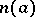satisfying the following requirement: For any two elementsandwith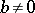one can find elementsandsuch that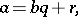where either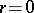or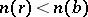.
Every Euclidean ring is a principal ideal ring and hence a factorial ring; however, there exist principal ideal rings that are not Euclidean. Euclidean rings include the ring of integers (the absolute value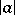plays the part of), and also the ring of polynomials in one variable over a field (is the degree of the polynomial). In any Euclidean ring the Euclidean algorithm can be used to find the greatest common divisor of two elements.